Equation Editor
•  714,894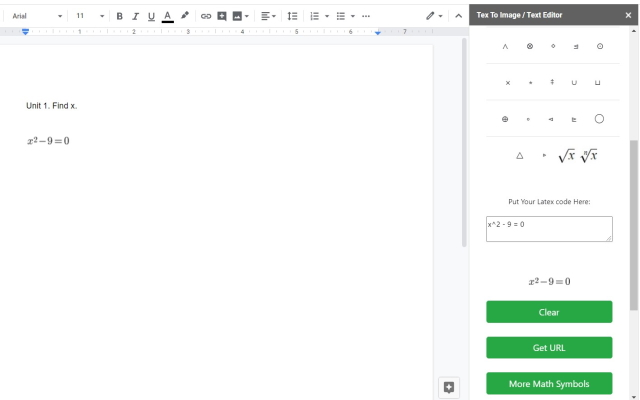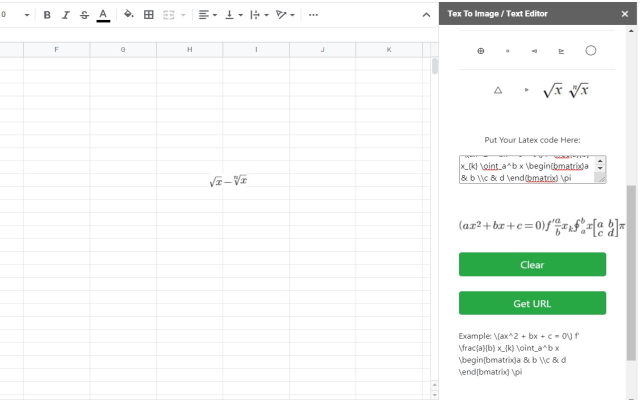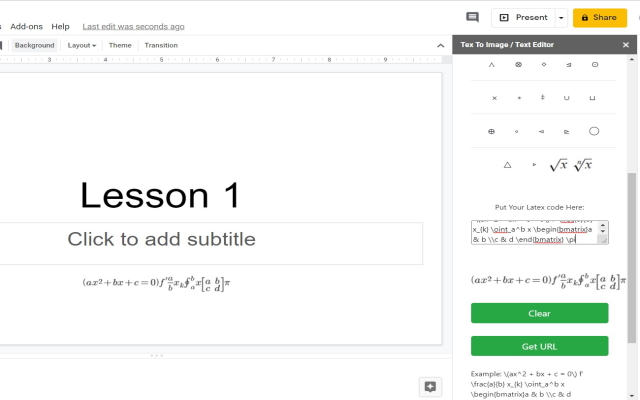Overview
Edit and add in math formulas inside Google Documents/Google Slides. Write math formulas for homework, exams and teaching materials.
```Our app is a free online add on to add and edit math formulas. You need to prepare for for your studies, exams and teaching materials.

Ever wanted a simple method to write math equations without having to use another app/software? Our addon will help you to write math equations easily on the computer.

You can add and edit math formulas very simply and intuitively, then save the output file as png file format and insert this math equation image to your Google Docs/Google Slides.

To begin using the software, simply do the following. Click on Addons - Equation Editor for Maths - and our addon sidebar will open up. Choose the math symbols you need to use to begin writing out your math equation. Once you have finished writing out your math equation, press the save button and our software will save this math equation as an image.
Common Math Symbols which are provided include:
Greater then or equal to
Square Root
To the power of
Fractions
and much more...

This is a lite, free & flexible LaTeX editor app for your Google Documents, Slide, Sheet.```
Reviews
Language:
Sort by: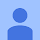A User of Equation Editor for Maths
August 8, 2020
i'd give it a zero if i could. don't waste your time.
Yes
No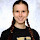Jaime Krause
August 7, 2020
Same: Doesn't work
Yes
NoA User of Equation Editor for Maths
August 3, 2020
Yes
NoA User of Equation Editor for Maths
August 3, 2020
As the other reviews stated, the add on isn't working. When going to the add on menu to choose it, the only thing that is displaying is the help option. It seem to be a great concept, but needs to work in order for me to utilize it.
Yes
No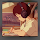A User of Equation Editor for Maths
August 2, 2020
doesnt work
Yes
NoA User of Equation Editor for Maths
July 30, 2020
Doesn't work.
Yes
No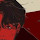A User of Equation Editor for Maths
July 28, 2020
not working
Yes
NoA User of Equation Editor for Maths
July 27, 2020
didn't work